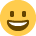# A sum for you!

Well-KnownRegistered Posts: 225
5 + 5 + 5 = 550

You can draw one straight line to make the sum correct.

• Yazi wrote: »
5 + 5 + 5 = 550

You can draw one straight line to make the sum correct.

[strike]5+5+5=550[/strike]

It makes me really sad that I can't use [strike].

That would've been THE best answer
• Experienced Mentor Registered Posts: 591
If you put a strike through the equals sign then it changes it to the mathematical symbol for "does not equal", so i suppose that would work.....
• Font Of All Knowledge Registered Posts: 1,954
uknitty wrote: »
If you put a strike through the equals sign then it changes it to the mathematical symbol for "does not equal", so i suppose that would work.....

Technically right!• Put a diagonal / on the first plus sign to change it to 545 + 5 = 550